Next: Amplitude corrections for pegleg Up: Particular Implementation of LSJIMP Previous: Particular Implementation of LSJIMP

## Kinematic imaging of pegleg multiples in a laterally-homogeneous earth

In a 1-D Earth'' (horizontally-stratified, v(z) medium), the normal-moveout (NMO) equation Taner and Koehler (1969) describes the prestack traveltime curve of a primary reflection at relatively short source-receiver offset: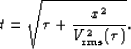(16)
Applied as an offset-dependent vertical time shift to a CMP gather, the NMO equation flattens an arbitrary primary to its zero-offset traveltime, where (half) offset is denoted x and the root-mean-square (RMS) velocity,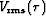, is defined in a laterally-homogeneous earth as: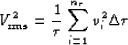(17)
The earth is parameterized bylayers of time thickness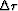, with constant interval velocity vi in each layer.

Analogously, a modified NMO equation can image pegleg multiples in a 1-D Earth, as motivated graphically by Figure. From the figure, we see that kinematically, a first-order pegleg can be conceptualized as a pseudo-primary'' with the same offset, but with an additional two-way zero-offset traveltime to the multiple generator,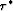. In equation form, let us extend this intuition to the general case of a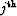-order pegleg to write an NMO equation for peglegs:(18)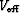is the effective RMS velocity of the pseudo-primary shown in Figure. To derive an expression for, we modify the definition of RMS velocity, equation (), to reflect a-order pegleg multiple's additional travel between the surface and multiple generator:(19)
Analogously,is the number of assumed layers between the earth's surface and the multiple generator. Notice that the two terms inside the parentheses of equation () are simply the definition of RMS velocity atand, respectively. We can substitute equation () accordingly to derive the final expression for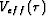:(20)
Wang (2003) derives a similar series of expressions.

 schem Figure 3 Pegleg multiples S201G'' and S102G'' have the same traveltimes as pseudo-primary'' with the same offset and an extra zero-offset traveltime.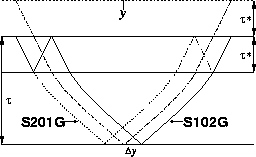Next: Amplitude corrections for pegleg Up: Particular Implementation of LSJIMP Previous: Particular Implementation of LSJIMP
Stanford Exploration Project
5/30/2004